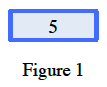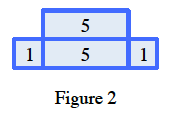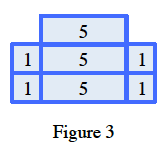Home > CC3MN > Chapter cc35 > Lesson cc35.1.1 > Problem5-5

5-5.

A tile pattern has $5$ tiles in Figure $0$ and adds $7$ tiles in each new figure. Write the equation of the line that represents the growth of this pattern.   5-5 HW eTool (Desmos). Homework Help ✎

Illustrate the problem.

Use the equation of a line to substitute in known information.

$y=mx+b$

$m=$ the rate of growth between each figure
$b=$ the starting number of tiles

$y=7x+5$Use the eTool below to help you with this problem.
Click on the link at right for the full eTool version: CC3 5-5 HW eTool